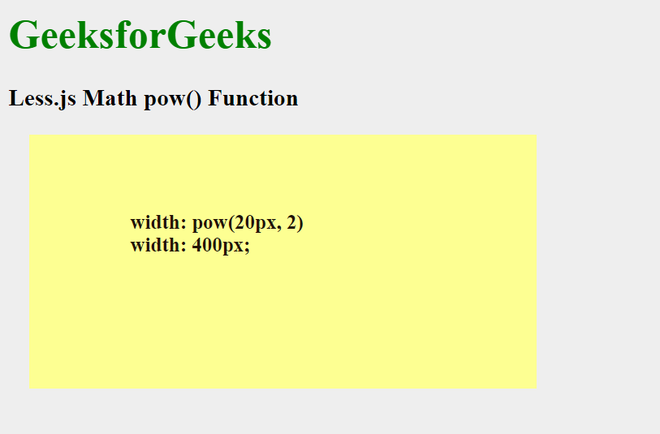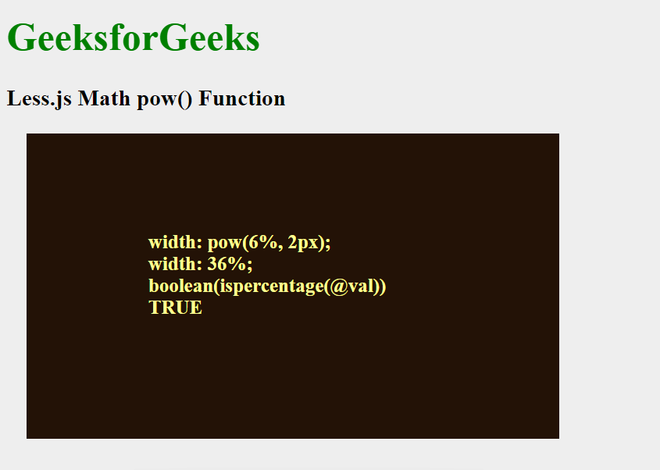GFG App
Open AppBrowser
Continue

Less.js is a simple CSS pre-processor that facilitates the creation of manageable, customizable, and reusable style sheets for websites. It is chosen because CSS is a dynamic style sheet language. LESS is flexible, thus it functions with a variety of browsers. Web browsers can only use CSS that has been written in and processed using the CSS pre-processor, a programming language. It is a CSS language extension that also offers features like variables, functions, mixins, and operations to aid in the creation of dynamic CSS while keeping backward compatibility.

In this article, we are going to discuss the Math pow() function, which is used to return the first argument’s value rounded up to the power of the second argument. This function takes two floating numbers as parameters and performs a to the power operation keeping the first number as a base and the second number as an exponent. The returned values have the same dimension as the first parameter and the dimension of the second parameter is ignored.

Syntax:

`power(number, number)`

Parameters:

• number: This is the first compulsory parameter for this function. This parameter takes a floating number and this is the base for the power operation.
• number: This is the second compulsory parameter for this function. This parameter takes a floating number and this is the exponent for the power operation.

Compile LESS code into CSS code.

Example 1: The code below demonstrates the usage and implementation of the Misc pow() function.

## HTML

 ` ` `<``html``> ` ` `  `<``head``> ` `    ``<``link` `rel``=``"stylesheet"` `type``=``"text/css"`  `           ``href``=``"style.css"``/> ` ` ` ` `  `<``body``> ` `    ``<``h1` `style``=``"color:green"``>GeeksforGeeks   ` `    ``<``h3``><``b``>Less.js Math pow() Function ` `    ``<``div` `class``=``"c1"``>   ` `        ``<``p` `class``=``"p1"``> ` `            ``<``strong``> ` `                ``width: pow(20px, 2) ` `                ``<``br``> width: 400px; ` `            `` ` `        `` ` `    `` ` ` ` ` `  ``

styles.less:

## CSS

 `@body-bg-``color``: ``#eeeeee``; ` `@dark: hsl(``25``, ``71%``, ``8%``); ` `@light: ``rgb``(``253``, ``255``, ``146``); ` `@val: pow(``20px``, ``2``); ` `body { ` `    ``background-color``: @body-bg-color; ` `} ` `.c``1` `{ ` `    ``width``: @val; ` `    ``height``: calc(@val/``2``); ` `    ``margin``: ``1``rem; ` `    ``background-color``: @light; ` `} ` `.p``1` `{ ` `    ``padding``: ``60px` `0px` `0px` `80px``; ` `    ``color``: @dark; ` `}`

Now, to compile the above LESS code to CSS code, run the following command:

`lessc styles.less styles.css`

The compiled CSS file comes to be:

styles.css:

## CSS

 `body { ` `    ``background-color``: ``#eeeeee``; ` `} ` `.c``1` `{ ` `    ``width``: ``400px``; ` `      ``height``: calc(``400px``/``2``); ` `      ``margin``: ``1``rem; ` `      ``background-color``: ``#fdff92``; ` `} ` `.p``1` `{ ` `      ``padding``: ``60px` `0px` `0px` `80px``; ` `      ``color``: hsl(``25``, ``71%``, ``8%``); ` `}`

Output:Example 2: The code below demonstrates the usage and implementation of the Misc pow() function and the code shows how the dimensions of the first parameter is the dimension of the returned value. We have also used the ispercentage() and the if() and boolean logical functions.

## HTML

 ` ` `<``html``> ` ` `  `<``head``> ` `    ``<``link` `rel``=``"stylesheet"` `type``=``"text/css"`  `          ``href``=``"styles.css"``/> ` ` ` ` `  `<``body``> ` `    ``<``h1` `style``=``"color:green"``>GeeksforGeeks   ` `    ``<``h3``><``b``>Less.js Math pow() Function ` `    ``<``div` `class``=``"c1"``>   ` `        ``<``p` `class``=``"p1"``> ` `            ``<``strong``> ` `                ``width: pow(6%, 2px);<``br``> ` `                ``width: 36%;<``br``> ` `                ``boolean(ispercentage(@val)) ` `                ``<``br``> TRUE ` `            `` ` `        `` ` `    `` ` ` ` ` `  ``

styles.less:

## CSS

 `@body-bg-``color``: ``#eeeeee``; ` `@dark: hsl(``25``, ``71%``, ``8%``); ` `@light: ``rgb``(``253``, ``255``, ``146``); ` `@val: pow(``6%``, ``2px``); ` `@cond: boolean(ispercentage(@val)); ` `body { ` `    ``background-color``: @body-bg-color; ` `} ` `.c``1` `{ ` `    ``width``:  @val; ` `    ``height``: ``250px``; ` `    ``margin``: ``1``rem; ` `    ``background-color``: if(@cond, @dark, @light); ` `} ` `.p``1` `{ ` `    ``padding``: ``80px` `0px` `0px` `100px``; ` `    ``color``: if(@cond, @light, @dark); ` `}`

Now, to compile the above LESS code to CSS code, run the following command:

`lessc styles.less styles.css`

The compiled CSS file comes to be:

styles.css:

## CSS

 `body { ` `    ``background-color``: ``#eeeeee``; ` `} ` `.c``1` `{ ` `    ``width``: ``36%``; ` `      ``height``: ``250px``; ` `      ``margin``: ``1``rem; ` `      ``background-color``: hsl(``25``, ``71%``, ``8%``); ` `} ` `.p``1` `{ ` `      ``padding``: ``80px` `0px` `0px` `100px``; ` `      ``color``: ``#fdff92``; ` `}`

Output:My Personal Notes arrow_drop_up